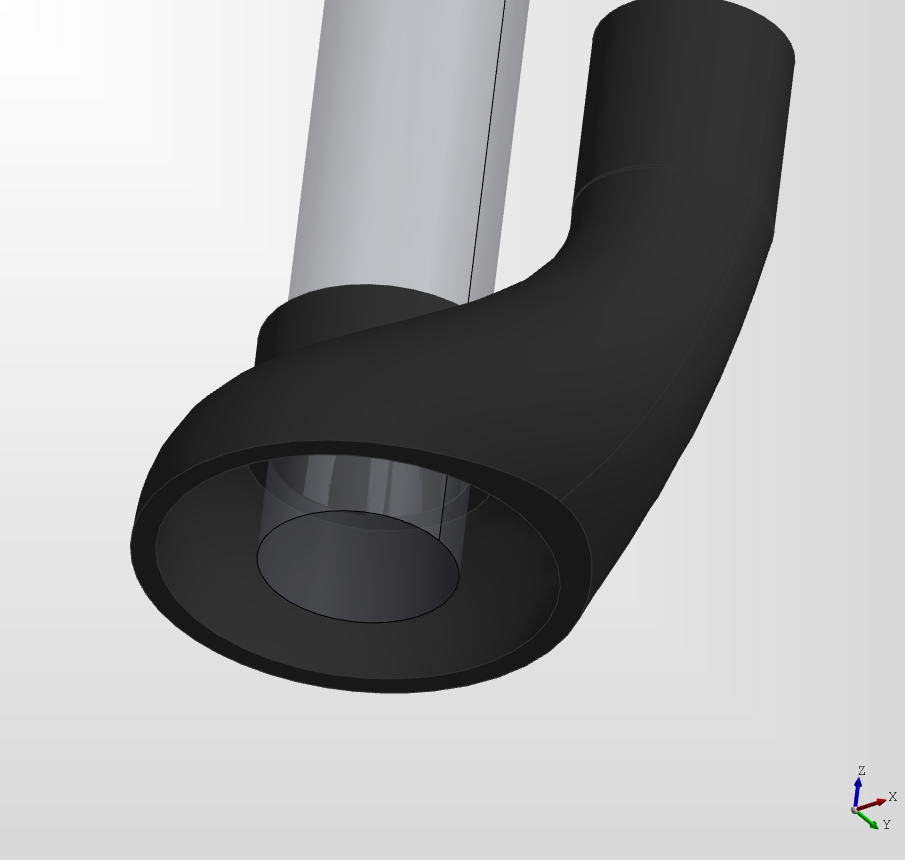## Lofts

In DeclaraCAD the `ThruSections` declaration is used to perform a loft as it follows OpenCASCADE naming conventions. As the name implies the "through sections" it lets you create a shape by lofting through several cross sections of the shape. Each child shape will be used as a section of the loft.

Note: Use `from declaracad.occ.api import ThruSections as Loft` to rename it

To create a loft first create the sections to loft between. Here three circles are created oriented at different positions and heights.

``````# Created in DeclaraCAD

enamldef Assembly(Part):
attr thickness = 0.2
attr diameter = 3

Wire:
color = 'red'
Circle: c1:
Wire:
color = 'red'
Circle: c2:
position << (c1.x+3,c1.y,c1.z+5)
direction = (1,0,0)

Wire:
color = 'red'
Circle: c3:
position << (c1.x+6,c1.y,c1.z+3)
direction = (0,0,-1)

# For reference
Line: xaxis:
color = 'black'
direction = (1, 0)
Line: yaxis:
color = 'black'
direction = (0, 1)
Line: zaxis:
color = 'black'
direction = (0, 0, 1)
``````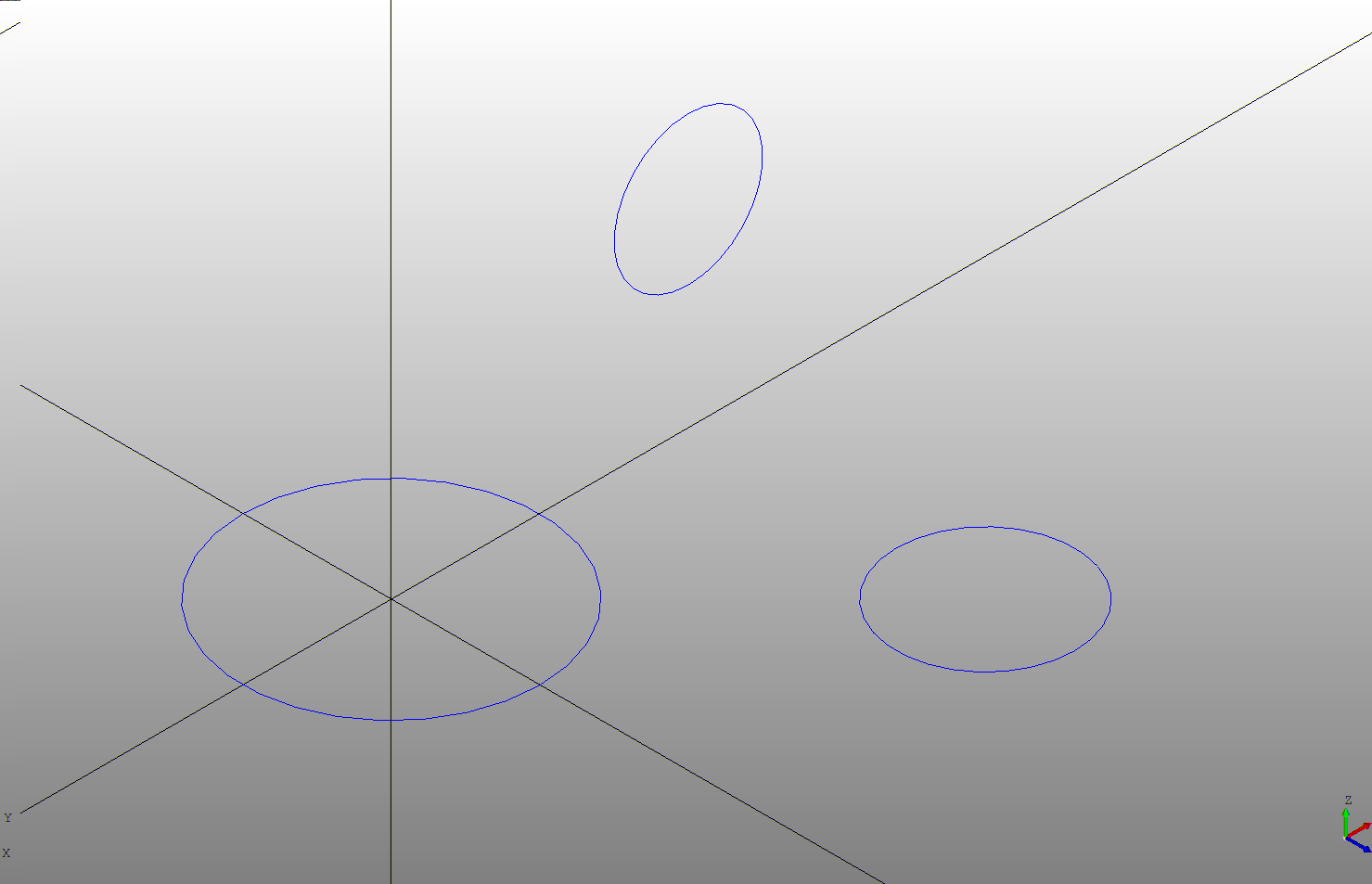Next add the cross sections as children of the `ThruSections`

``````# Created in DeclaraCAD

enamldef Assembly(Part):
attr thickness = 0.2
attr diameter = 3

ThruSections:
material = 'chrome'
Wire:
Circle: c1:
Wire:
Circle: c2:
position << (c1.x+3,c1.y,c1.z+5)
direction = (1,0,0)

Wire:
Circle: c3:
position << (c1.x+6,c1.y,c1.z+3)
direction = (0,0,-1)

# For reference
Line: xaxis:
color = 'black'
direction = (1, 0)
Line: yaxis:
color = 'black'
direction = (0, 1)
Line: zaxis:
color = 'black'
direction = (0, 0, 1)
``````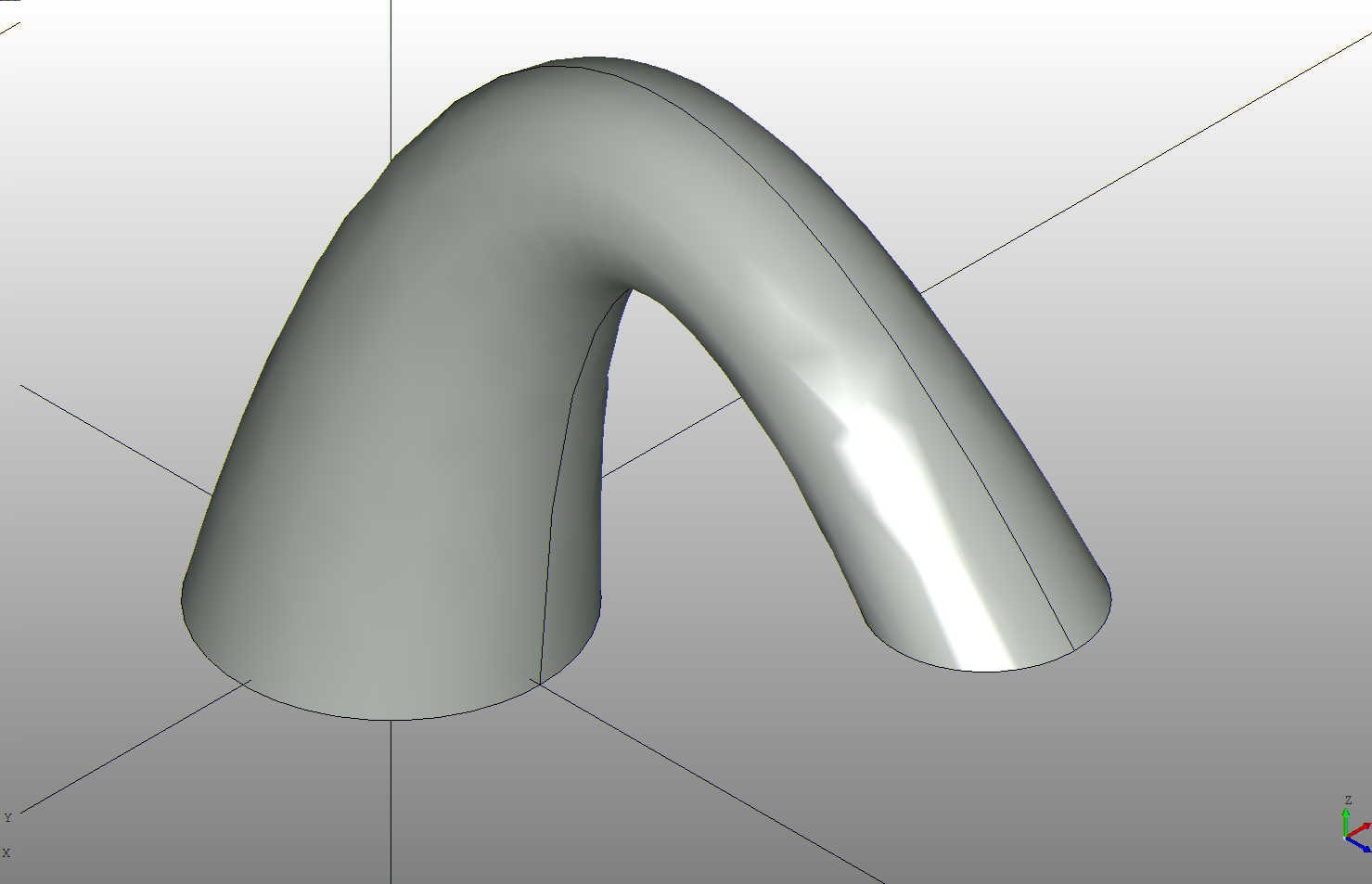Since the cross sections used are wires and not faces it creates a hollow surface as shown below.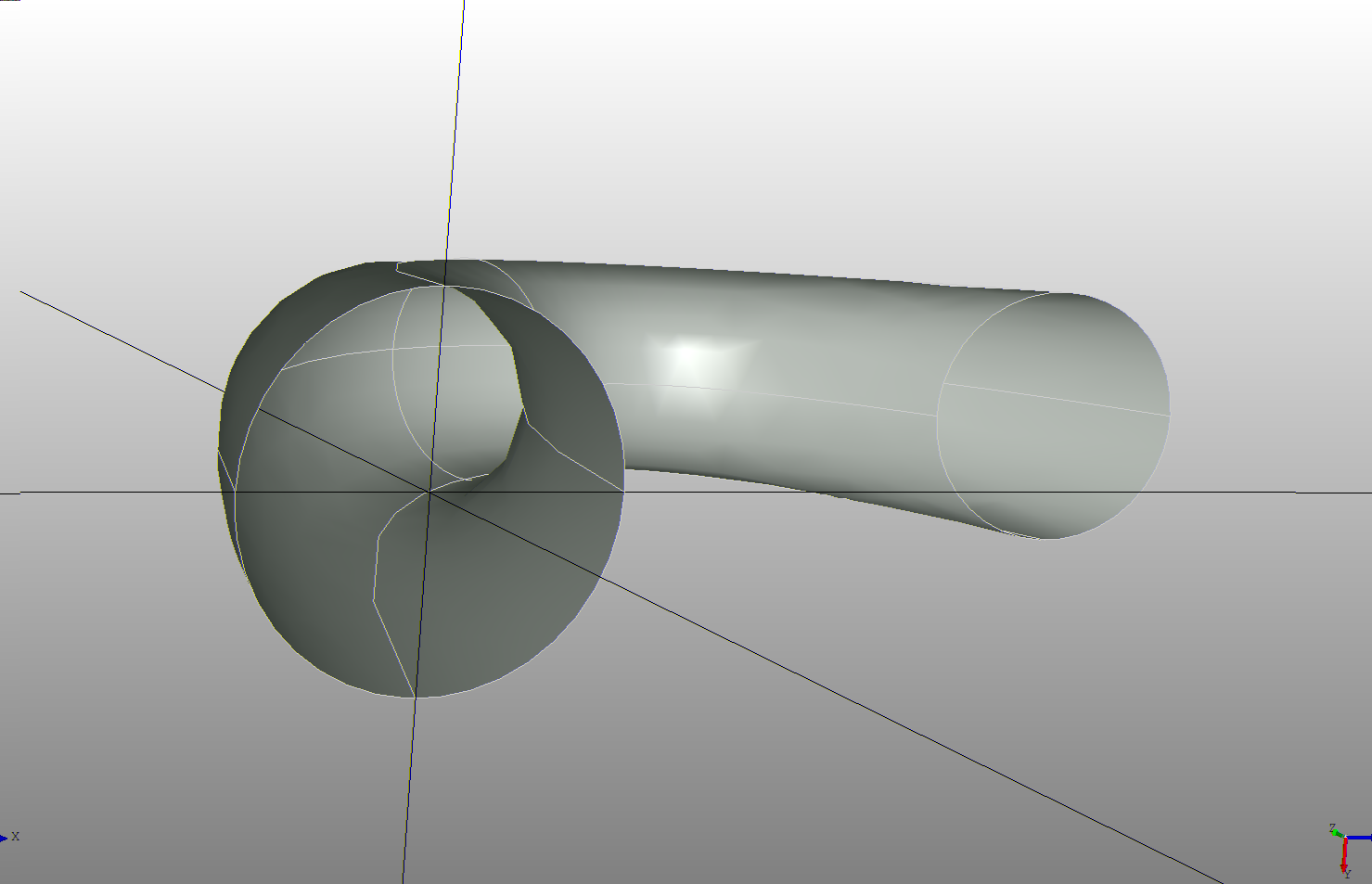To make it a solid set `solid = True` which will fill in the sections.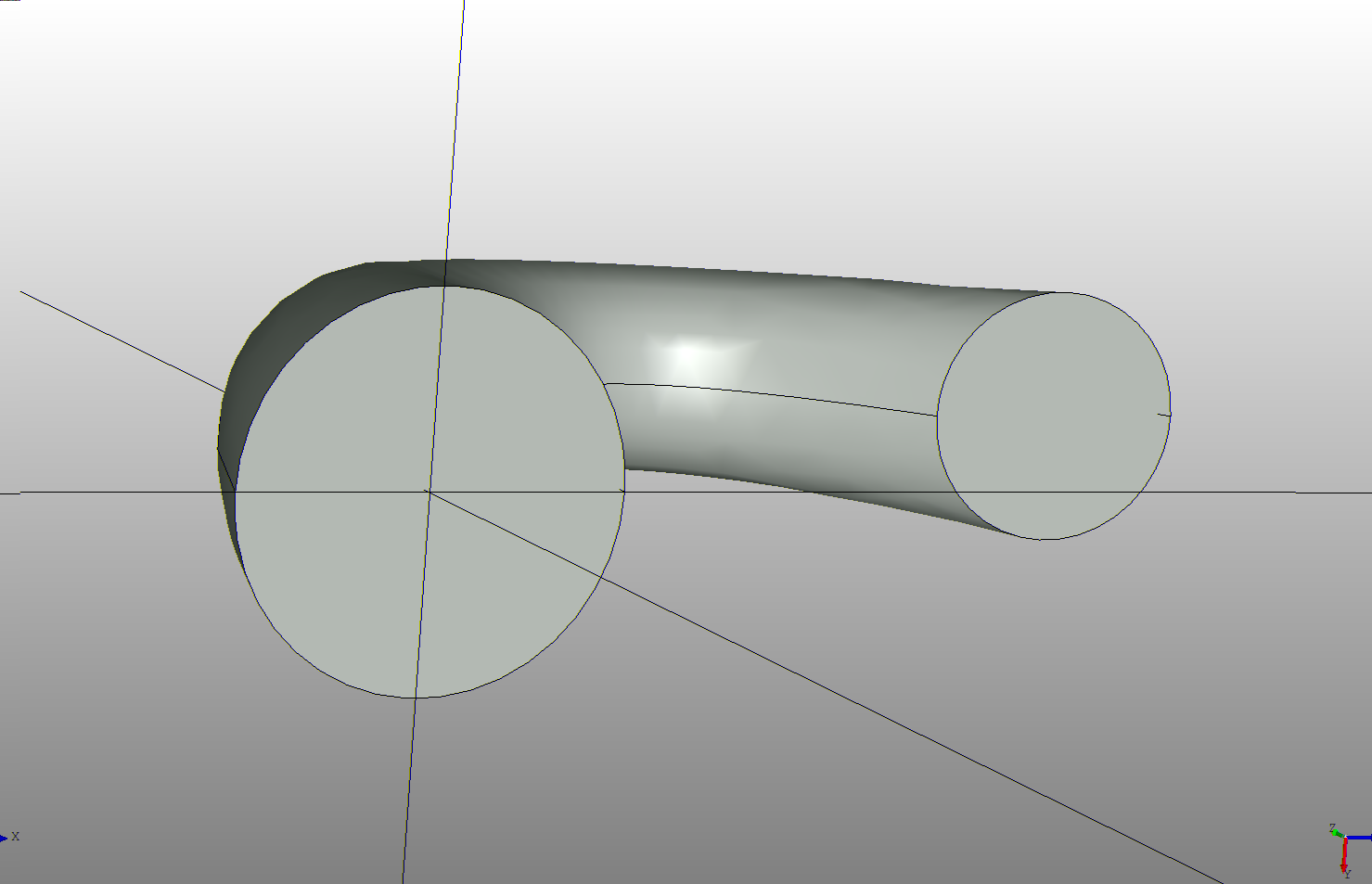By default the loft will generate smooth curves between each of the sections. If this is not desired set `ruled = True` which changes them to straight lines as shown below.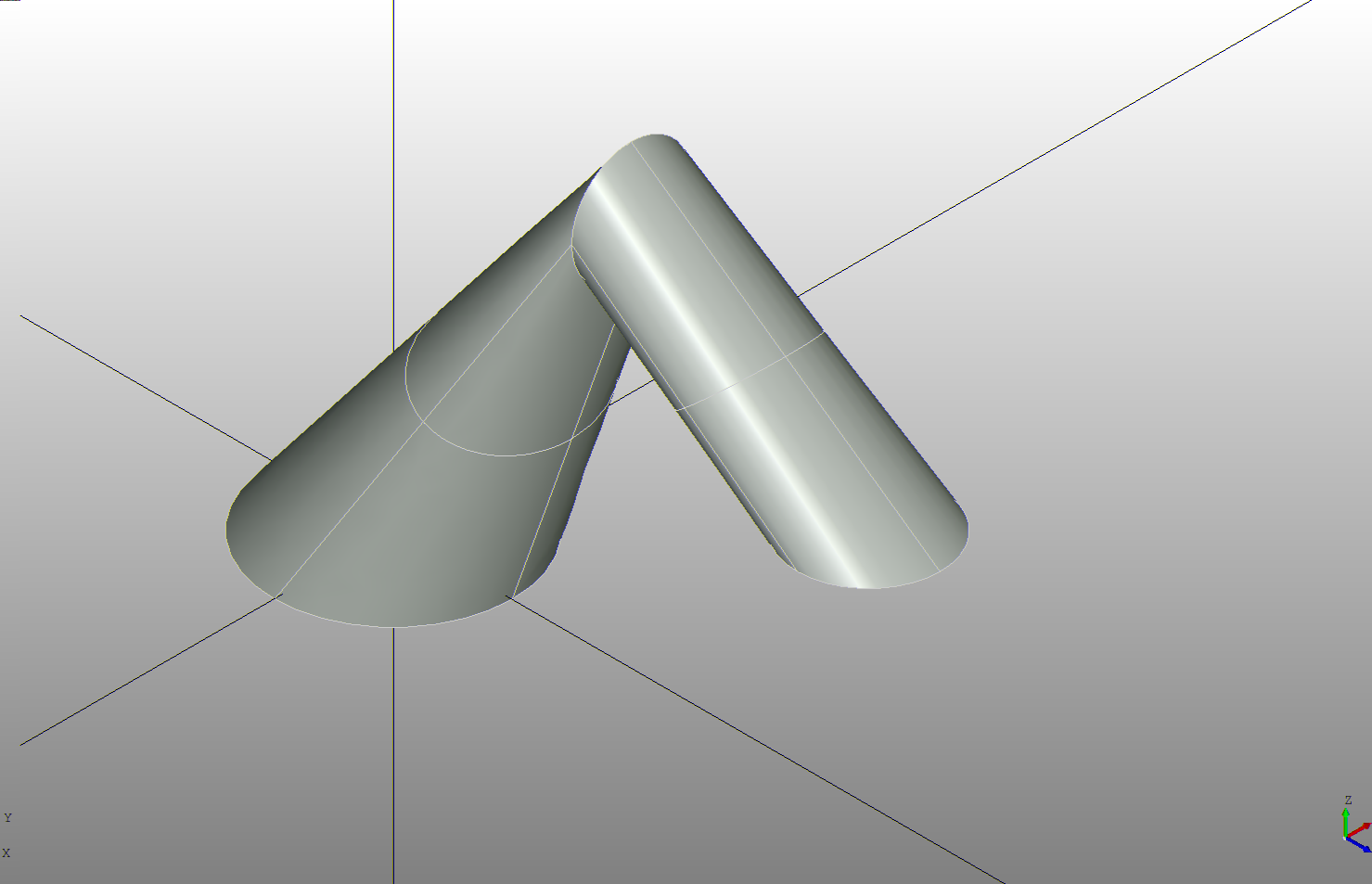### Example

Lofts can be fused with other shapes to create more complex parts such as this spindle vacuum nozzle.